# Tutorial: Define an OML Custom Block

Learn how to define the behavior of a spring damper model using an OML Custom Block.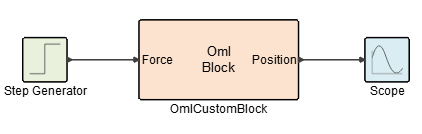## Files for This Tutorial

Spring_Damper_oml_block.scm

You can access a finished version of the model that you build in this tutorial from the Demo Browser: Activate > Custom Blocks > Spring_Damper_oml_block.scm or product installation: <installation_directory>/_demos/Custom Blocks/Spring_Damper_oml_block.scm.

## Create the Spring Damper Model

Create a simple spring damper model with an OML Custom Block, Step Generator and Scope block. Define the Context of the model with four parameters, F, M, k_s and k_d, that represent the applied force, mass, stiffness and damping of the system, respectively, and that are called through the simulation function of the OML Custom Block.
1. Drag an OML Custom Block, Step Generator and Scope block into your model diagram:
• Palettes > Activate > CustomBlocks > OMLCustomBlock
• Palettes > Activate > Signal Generators > StepGenerator
• Palettes > Activate > SignalViewers > Scope3. Save and name your model.
4. Select the Diagram icon.5. Define the Context of the system model where F is the applied force, M is mass, k_s is the spring coefficient, k_d is the damping coefficient, and x0 specifies the initial state for velocity and position:
F=2;
M-2;
k_s = 1;
k_d = 1;
x0 = [0,0];

## Define the Step Generator

1. Double-click the Step Generator block.
2. For Final value, enter F, which is the Force.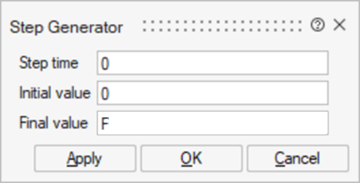3. Keep the default values for the Step time and Initial value.

## Define the OML Custom Block

Define the ports, states, parameters, and simulation function for the OML Custom Block.

1. Double-click the Oml Custom Block in your model.
2. Select the Ports tab.
• For Number of input ports, enter 1 and name it Force.
• Select the Feedthrough option, which specifies that the system input directly affects the output.
• Enter 1 output port and name it Position.
• Keep the defaults for the remaining fields.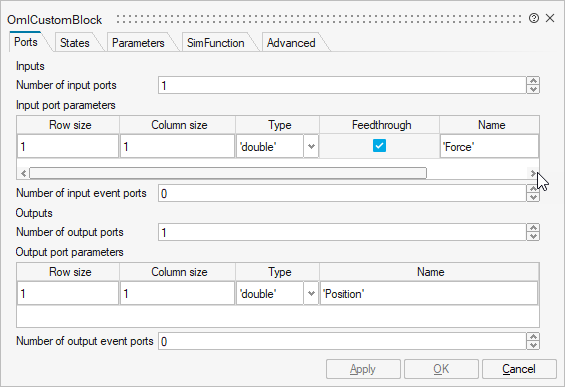3. Select the States tab:
• For the Initial continuous state, enter x0.
• Keep the defaults for the remaining fields.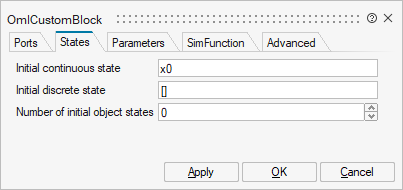4. Select the Parameters tab:
For Number of object parameters, enter 4 and define them as follows:

### Define the Sim Function

1. Select the SimFunction tab.
2. Click the generate skeleton button, then click the text editor button.
The software generates a code skeleton with the appropriate flag options based on the information you defined in the simulation function and diagram context. You utilize the flags that you want to include and ignore the flags that are not required for your model.
Note: The structure of the skeleton that is generated is the same for different custom blocks, such as CCustomBlock and PyCustomBlock, respecting the syntax of each programming language that is deployed.
3. To complete the simulation function for the spring damper, modify the code in 4 places as you see in the following image:
1. Line 9, add: Position = x(2);
2. Line 17, uncomment:
vssSetOutputData (block,1, Position, vssGetOutputDataType (block,1));
3. Line 20, add the equation of motion:
xd = [(Force-k_d*x(1)-k_s*x(2))/M ; x(1)];
4. Line 21, uncomment
vssSetDerState(block,<derivative value>);
and modify <derivative value> to reference the drivative state variable:
vssSetDerState(block,xd);
Your new script should look like this: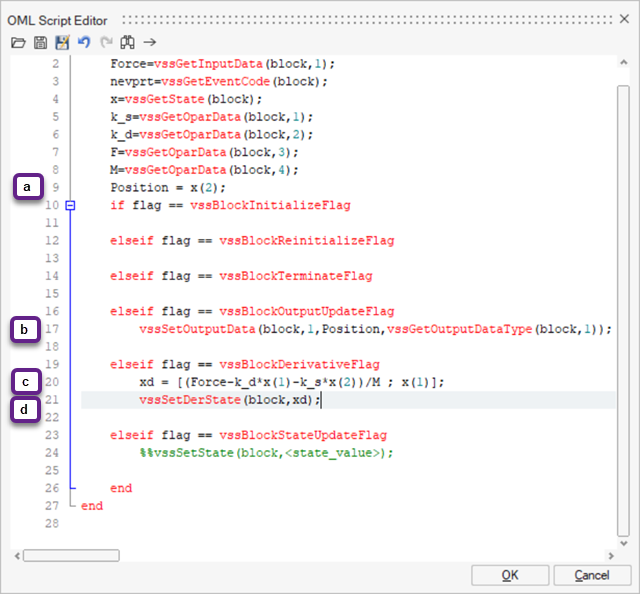4. Click OK.
For your reference, the following table explains the flags presented in the skeleton for this tutorial.
Flag/API Description Applied in this tutorial
Force=vssGetInputData(block,1); vssGetInputData is called to define the Force variable as equal to the block's signal input. Yes
nevprt=vssGetEventCode(block);  GetEventCode is called to define the variable nevprt as equal to the block's event input (none in this case). Yes
x=vssGetState(block); GetState is called to define the variable x as equal to the given block states. Yes
k_s=vssGetOparData(block,1);  GetOparData is called to define the the variable k_s as equal to the given Object Parameter 1 that is defined in the Parameters tab. Yes
k_d=vssGetOparData(block,2); GetOparData is called to define the the variable k_d as equal to the given Object Parameter 2, which is defined in the Parameters tab. Yes
F=vssGetOparData(block,3); GetOparDatais called to define the variable F as equal to the given Object Parameter 3, which is defined in the Parameters tab. Yes
M=vssGetOparData(block,4); GetOparData is called to define the the variable M as equal to the given Object Parameter 4, which is defined in the Parameters tab. The API is also called to enable the calculation of Position = x(2); Yes
if flag == vssBlockInitializeFlag This flag is called at the start of a simulation to allocate memory, or to open a file or channel. No
elseif flag == vssBlockReinitializeFlag  This flag is used mainly with continuous blocks for updating states based on a defined input. For example, the block can be called by the simulator during the initialization phase to find the steady state of a system. No
elseif flag == vssBlockTerminateFlag This flag is called only once at the end of a simulation if the block requires final tasks such as clearing variables, exporting, or organizing information once the block execution has finished. No
elseif flag == vssBlockOutputUpdateFlag This flag is called to compute the outputs during a simulation and is called every time the block is activated. In this tutorial, the Output that is triggered is defined with vssSetOuputData. Yes
 vssSetOutputData (block,1,Position,vssGetOutputDataType(block,1)); vssSetOutputData is called to define the output. Yes
vssGetOutputDataType is is called to assign a data type to the output signal. Yes
elseif flag == vssBlockDerivativeFlag

xd = [(Force-k_d*x(1)-k_s*x(2))/M ; x(1)];

vssSetDerState(block,xd);
This flag is called to apply a computation to the system, which in this tutorial is the equation of motion (xd = [(Force-k_d*x(1)-k_s*x(2))/M ; x(1)];). The API vssSetDerState is then called to set the derivative state equal to the system's velocity that was solved in the equation of motion. Yes
elseif flag == vssBlockStateUpdateFlag  This flag is called for the blocks that have states to update the states. This flag is called every time that a block is activated. No

2. For Activation mode, select Always Active mode, which specifies that the block is dependent on time and will be called continuously at each step of the simulation.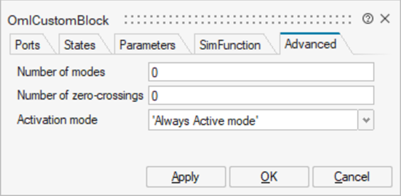3. Click OK.
The setup for the spring damping model is complete.

### Run the Simulation

1. Keep the default values for the simulation parameters.
2. Click Run.3. When the simulation finishes running, double-click the Scope block.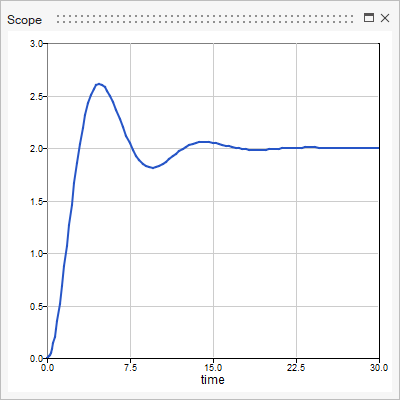The scope shows the signal output from the OML Custom Block as Position vs. Time.